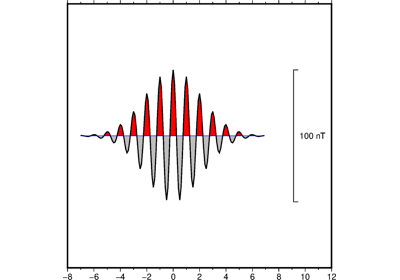# pygmt.Figure.wiggle¶

Figure.wiggle(x=None, y=None, z=None, data=None, *, frame=None, position=None, color=None, projection=None, region=None, track=None, timestamp=None, verbose=None, pen=None, xshift=None, yshift=None, scale=None, panel=None, columns=None, perspective=None, **kwargs)

Plot z=f(x,y) anomalies along tracks.

Takes a matrix, (x,y,z) triplets, or a file name as input and plots z as a function of distance along track.

Must provide either `data` or `x`/`y`/`z`.

Full parameter list at https://docs.generic-mapping-tools.org/latest/wiggle.html

Aliases:

• B = frame

• D = position

• G = color

• J = projection

• R = region

• T = track

• U = timestamp

• V = verbose

• W = pen

• X = xshift

• Y = yshift

• Z = scale

• c = panel

• i = columns

• p = perspective

Parameters
• x/y/z (1d arrays) – The arrays of x and y coordinates and z data points.

• data (str or numpy.ndarray or pandas.DataFrame or xarray.Dataset or geopandas.GeoDataFrame) – Pass in either a file name to an ASCII data table, a 2D `numpy.ndarray`, a `pandas.DataFrame`, an `xarray.Dataset` made up of 1D `xarray.DataArray` data variables, or a `geopandas.GeoDataFrame` containing the tabular data. Use parameter `columns` to choose which columns are x, y, z, respectively.

• projection (str) – Required if this is the first plot command. projcode[projparams/]width. Select map projection.

• region (str or list) – Required if this is the first plot command. xmin/xmax/ymin/ymax[+r][+uunit]. Specify the region of interest.

• scale (str or float) – Gives anomaly scale in data-units/distance-unit. Append c, i, or p to indicate the distance unit (cm, inch, or point); if no unit is given we use the default unit that is controlled by PROJ_LENGTH_UNIT.

• frame (bool or str or list) – Set map boundary frame and axes attributes.

• position (str) – [g|j|J|n|x]refpoint+wlength[+jjustify][+al|r][+odx[/dy]][+l[label]]. Defines the reference point on the map for the vertical scale bar.

• color (str) – Set fill shade, color or pattern for positive and/or negative wiggles [Default is no fill]. Optionally, append +p to fill positive areas (this is the default behavior). Append +n to fill negative areas. Append +n+p to fill both positive and negative areas with the same fill. Note: You will need to repeat the color parameter to select different fills for the positive and negative wiggles.

• track (str) – Draw track [Default is no track]. Append pen attributes to use [Default is 0.25p,black,solid].

• timestamp (bool or str) – Draw GMT time stamp logo on plot.

• verbose (bool or str) –

Select verbosity level [Default is w], which modulates the messages written to stderr. Choose among 7 levels of verbosity:

• q - Quiet, not even fatal error messages are produced

• e - Error messages only

• w - Warnings [Default]

• t - Timings (report runtimes for time-intensive algorithms);

• i - Informational messages (same as `verbose=True`)

• c - Compatibility warnings

• d - Debugging messages

• pen (str) – Specify outline pen attributes [Default is no outline].

• xshift (str) – [a|c|f|r][xshift]. Shift plot origin in x-direction.

• yshift (str) – [a|c|f|r][yshift]. Shift plot origin in y-direction. Full documentation is at https://docs.generic-mapping-tools.org/latest/gmt.html#xy-full.

• panel (bool or int or list) – [row,col|index]. Selects a specific subplot panel. Only allowed when in subplot mode. Use `panel=True` to advance to the next panel in the selected order. Instead of row,col you may also give a scalar value index which depends on the order you set via `autolabel` when the subplot was defined. Note: row, col, and index all start at 0.

• columns (str or 1d array) – Choose which columns are x, y, and z, respectively if input is provided via data. E.g. `columns = [0, 1, 2]` or `columns = "0,1,2"` if the x values are stored in the first column, y values in the second one and z values in the third one. Note: zero-based indexing is used.

• perspective (list or str) – [x|y|z]azim[/elev[/zlevel]][+wlon0/lat0[/z0]][+vx0/y0]. Select perspective view and set the azimuth and elevation angle of the viewpoint. Default is [180, 90]. Full documentation is at https://docs.generic-mapping-tools.org/latest/gmt.html#perspective-full.

## Examples using `pygmt.Figure.wiggle`¶